# NCERT Solutions for Basic Geometrical Ideas

In this page we have NCERT Solutions for Class 6 Maths Basic Geometrical Ideas. Hope you like them and do not forget to like , social share and comment at the end of the page.

## Exercise 4.1

Question 1
Use the figure to name:
(a) Five points
(b) A line
(c) Four rays
(d) Five-line segments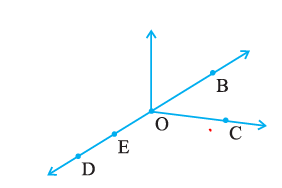Solution
 Point A point determines a location. It is usually denoted by a capital letter Line segment A line segment corresponds to the shortest distance between two points. The line segment joining points X and X is denoted by XY. Line A line segment extended on both side to indefinitely is called line Ray A ray is a portion of line starting at a point and going in one direction endlessly.
a) D, E, O,B,C
b)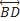c)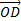,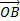,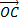,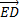d)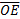,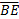,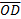,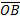,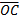Question 2
Name the line given in all possible (twelve) ways, choosing only two letters at a time from the four given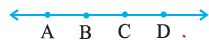Solution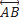,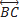,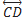,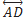,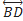,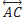,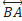,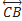,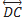,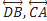,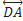,
Question 3
Use the figure to name:
(a) Line containing point E.
(b) Line passing through A.
(c) Line on which O lies
(d) Two pairs of intersecting lines.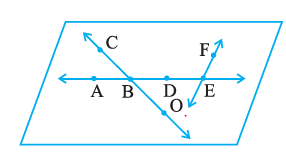Solution
a)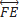b)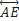c)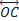d)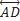andand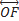Question 4
How many lines can pass through (a) one given point? (b) two given points?
Solution
(i) There are infinite lines which can pass through one point.
(ii) There is only one line which can pass through two given points.

Question 5
Draw a rough figure and label suitably in each of the following cases:
(a) Point P lies on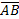(b)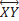and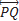intersect at M.
(c) Line l contains E and F but not D.
(d)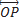and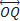meet at O.

Solution
a)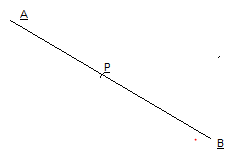b)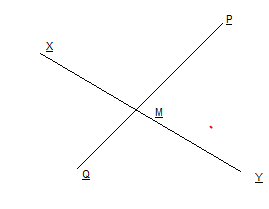c)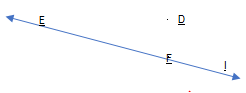d)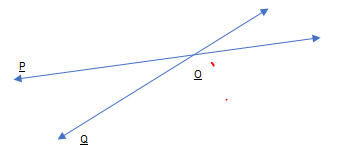Question 6
Consider the following figure of line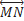Say whether following statements are true or false in context of the given figure.
(a) Q, M, O, N, P are points on the line.
(b) M, O, N are points on a line segment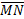.
(c) M and N are end points of line segment.
(d) O and N are end points of line segment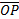.
(e) M is one of the end points of line segment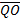.
(f) M is point on ray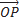(g) Ray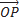is different from ray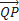(h) Rayis same as ray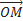(i) Rayis not opposite to ray(j) O is not an initial point of(k) N is the initial point of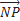and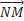.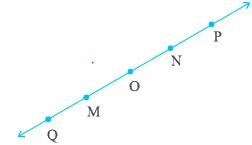Solution
(a) True
(b) True
(c) True
(d) False
(e) False
(f) False
(g) True
(h) False
(i) False
(j) False
(k) True

## Exercise 4.2

Question 1
Classify the following curves as (i) Open or (ii) Closed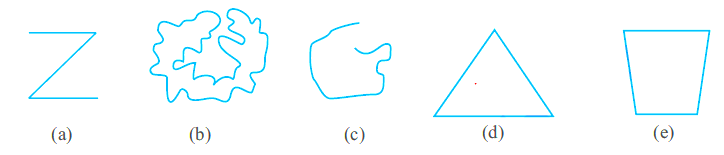Solutions

 Open A curve is called open if its ends are not joined Closed A curve is called close if its ends are joined
(a) Open
(b)Closed
(c)Open
(d)Closed
(e)Closed

Question 2
Draw rough diagrams to illustrate the following:
(a) Open curve (b) Closed curve.
Solution
a)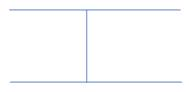b)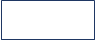Question 3
Draw any polygon and shade its interior.
Solution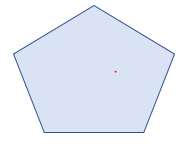Question 4
Consider the given figure and answer the questions:
(a) Is it a curve? (b) Is it closed?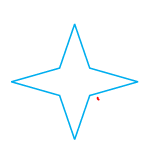Solution
a) it is curve
b) it is closed

Question 5.
Illustrate, if possible, each one of the following with a rough diagram:
(a) A closed curve that is not a polygon.
(b) An open curve made up entirely of line segments.
(c) A polygon with two sides.
Solution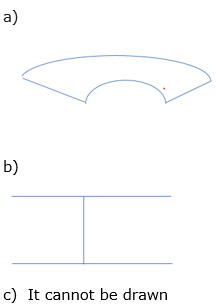## Exercise 4.3

Question 1
Name the angles in the given figure.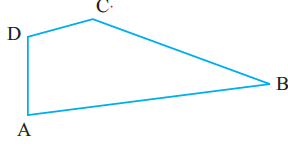Solution
$\angle DAB$, $\angle CBA$ , $\angle ADC$, $\angle DCB$

Question 2
In the given diagram, name the point(s)
(a) In the interior of $\angle DOE$
(b) In the exterior of $\angle EOF$
(c) On $\angle EOF$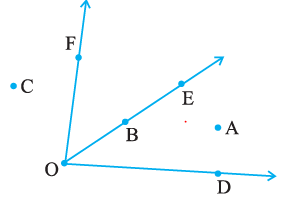Solution
(a) Point interior of $\angle DOE$ : A
(b) Points exterior of $\angle EOF$: C, A, D
(c) Points on $\angle EOF$: E, O, B, F
Question 3
Draw rough diagrams of two angles such that they have
(a) One point in common.
(b) Two points in common.
(c) Three points in common.
(d) Four points in common.
(e) One ray in common.
Solution
a)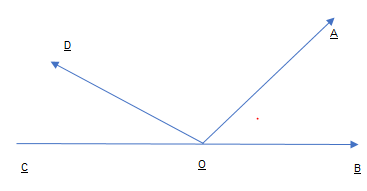O is the common point for two angles $\angle AOB$ and $\angle DOC$

b)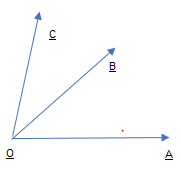O and B are  the common point for two angles  $\angle AOB$  and  $\angle BOC$

c)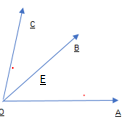O  , E and B are  the common point for two angles  $\angle AOB$ and  $\angle BOC$
d)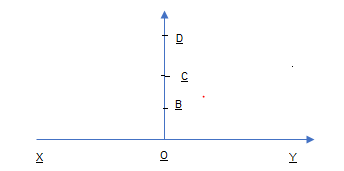O, B, C and D are common points for two angles  $\angle XOD$ and  $\angle YOD$
e)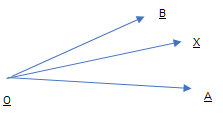OX is the ray common between the angle   $\angle BOX$  and  $\angle AOX$

## Exercise 4.4

Question 1
Draw a rough sketch of a triangle ABC. Mark a point P in its interior and a point Q in its exterior. Is the point A in its exterior or in its interior?
Solution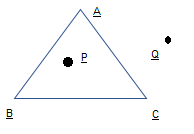Point A is neither interior nor exterior.  It is a vertex of the triangle
Question 2.
(a) Identify three triangles in the figure.
(b) Write the names of seven angles.
(c) Write the names of six-line segments.
(d) Which two triangles have ∠B as common?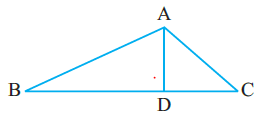Solution
a)  Triangles are ABD, ADC and ABC
b) $\angle ABD$ , $\angle ADB$, $\angle BAD$, $\angle DAC$, $\angle ACD$, $\angle ADC$
c) Line segments are AB, BD,AD,AC,DC,BC
d) ABC and ABD

## Exercise 4.5

Question 1
Draw a rough sketch of a quadrilateral PQRS. Draw its diagonals. Name them.
Is the meeting point of the diagonals in the interior or exterior of the quadrilateral?

Solution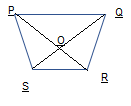POR and QOS are the diagonals
Their meeting point is interior
Question 2
Draw a rough sketch of a quadrilateral KLMN. State,
(a) two pairs of opposite sides,
(b) two pairs of opposite angles,
(c) two pairs of adjacent sides,
(d) two pairs of adjacent angles.
Solution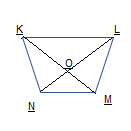a) KL and MN ,KN and ML
b)  $\angle NKL$ and   $\angle NML$  ,  $\angle KNM$ and $\angle KLM$
c) KN and NM, KL and LM
d)  $\angle NKL$ and  $\angle KLM$  ,  $\angle KNM$ and $\angle NML$

## Exercise 4.6

Question 1
From the figure, identify:
(a) the centre of circle
(c) a diameter
(d) a chord
(e) two points in the interior
(f) a point in the exterior
(g) a sector
(h) a segment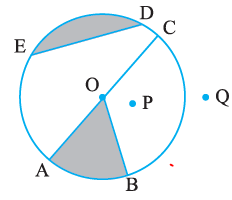Solution

 Circle A circle is the path of a point moving at the same distance from a fixed point Center Fixed point is called center Radius the fixed distance Chord A chord of a circle is a line segment joining any two points on the circle Diameter A diameter is a chord passing through the centre of the circle. Sector A sector is the region in the interior of a circle enclosed by an arc on one side and a pair of radii on the other two sides Segment A segment of a circle is a region in the interior of the circle enclosed by an arc and a chord.

(a) the centre of circle   O
(b) three radii OA, OB, OC
(c) a diameter AC
(d) a chord ED
(e) two points in the interior O and P
(f) a point in the exterior Q
(g) a sector OAB
(h) a segment ED

Question 2.
(a) Is every diameter of a circle also a chord?
(b) Is every chord of a circle also a diameter?
Solution
a) True
b) False

Question 3.
Draw any circle and mark
(a) its centre
(c) a diameter
(d) a sector
(e) a segment
(f) a point in its interior
(g) a point in its exterior
(h) an arc

Solution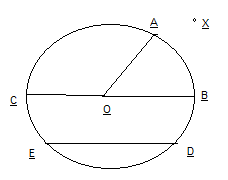a) Center O
c) Diameter BC
d) AOB is sector
e) ED is segment
f) O is point in it interior
h) X is the point exterior
g) AB is the arc
Question 4
Say true or false:
(a) Two diameters of a circle will necessarily intersect.
(b) The centre of a circle is always in its interior.
Solution
a) True
b) True

• NCERT Solutions
• Assignments

### Practice Question

Question 1 What is $\frac {1}{2} + \frac {3}{4}$ ?
A)$\frac {5}{4}$
B)$\frac {1}{4}$
C)$1$
D)$\frac {4}{5}$
Question 2 Pinhole camera produces an ?
A)An erect and small image
B)an Inverted and small image
C)An inverted and enlarged image
D)None of the above